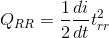# MCQs on Power Electronics

##### Page 8 of 10. Go to page 1 2 3 4 5 6 7 8 9 10
01․ A GTO can be turned on by applying
Positive gate signal.
Positive drain signal.
Positive source signal.
None of these.

Gate turn off (GTO), like an SCR is a device four layer, three junction semiconductor device with three external terminal (anode, cathode and gate). GTO can be turned on and off by positive pulse or signal and negative pulse or signal respectively to the gate terminal.

02․ SITH is also known as
Filled controlled diode.
Filled controlled rectifier.
Silicon controlled rectifier.
None of these.

They static induction thyristor (SITHs) is a thystor with a buried gate structure in which the gate electrodes are placed in n-base region. They are normally on-state, gate electrodes must be negatively biased to hold off-state. It is a self controlled GTO like device. Hence it is sometimes called field controlled diode.

03․ The reverse recovery time of diode is trr = 3 ÃŽÂ¼s and the rate off all of the diode current is di/dt = 30 A/ÃŽÂ¼s. The storage charge current QRR is
130 ÃŽÂ¼s.
135 ÃŽÂ¼s.
140 ÃŽÂ¼s.
145 ÃŽÂ¼s.04․ The turn-on time of an SCR with inductive load is 20 µs. The puls train frequency is 2.5 KHz with a mark/space ratio of 1/10, then SCR will
Turn on.
Not turn on.
Turn on if inductance is removed.
Turn on if pulse frequency us increased to two times.

Pulse repetition rate (PRR) = 1/(2.5 - 103) = 0.4 ms = 400 µs. Mark/space ratio = 1/10. Pulse width = 400/11 = 36.4 µs. The SCR will turn on because the pulse width is more than SCR turn on time.

05․ A power MOSFET has three terminals called
Collector, emitter and gate.
Drain, source and gate.
Drain, source and base.
Collector, emitter and base.

A power MOSFET has three terminals called drain, source and gate in place corresponding three terminals collector, emitter and base base for BJT.

06․ A modern power semiconductor device that combines the characteristic of BJT and MOSFET is
IGBT.
FCT.
MCT.
GTO.

IGBT process high input input impedance like a MOSFET and has on state power loss as in a BJT.

07․ Which one is most suitable power device for high frequency (>100 KHz) switching application?
BJT.
Power MOSFET.
Schottky diode.
Microwave transistor.

Power MOSFET has low turn off time. So it can be operated in a frequency range of 1 to 10 MHz.

08․ Thermal voltage VT can be given by
Kq/T.
KT/q.
qT/K.
(K2/q)(T + 1/T - 1).

The thermal voltage is given by VT = Kq/T Where, q = electron change, T = absolute temperature, K = Boltzman Constant.

09․ IGBT combines the advantages of
BJTs and SITs.
BJTs and MOSFETs.
SITs and MOSFETs.
None of these.

IGBT combines the advantage of BJTs and MOSFETs. IGBT process high input impedance like a MOSFET and has low on state power loss as in a BJT.

10․ COOLMOS device can be used in application up to power range of
1 KVA.
2 KVA.
500 VA.
100 KVA.

COOLMOS used in application up to power range of 2 KVA.

<<<678910>>>